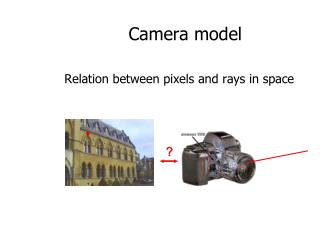DownloadDownload PresentationCamera model

# Camera model

Download Presentation## Camera model

- - - - - - - - - - - - - - - - - - - - - - - - - - - E N D - - - - - - - - - - - - - - - - - - - - - - - - - - -
##### Presentation Transcript

1. Camera model Relation between pixels and rays in space ?

2. Pinhole camera

3. Pinhole camera model Camera is a mapping between 3D world (object space) and 2D image; Camera model: matrix representing camera mapping; interested in central projection P = Intersection of principle axis With image plane Plane through camera Center C, parallel to image Plane is called principle Plane of the camera Line from camera Center perp. To image plane Focal plane Z=f linear projection in homogeneous coordinates!

4. Pinhole camera model

5. Principal point offset So far, assumed the origin of the coordinate in the image plane is the Same as the principal point principal point

6. Principal point offset calibration matrix

7. Camera rotation and translation Points in space are usually expressed in terms of world coordinate frame, i.e. Euclidean Inhomogeneous vector in world coordinate Coordinates of camera center in world coord Inhomogeneous 3 vector in camera coord. ~ General mapping for pinhole camera 9 deg of freedom: f, px,py,3 rot, 3 trans

8. CCD camera Pinhole camera model assumes image coordinates are Euclidean coordinates with equal scales in both axial directions; CCD cameras does not have square pixels mx is the number of pixels per unit distance in image coordinates in the x direction my is the number of pixels per unit distance in image coordinates in the y direction αx = f mx and αy = f my : focal length of the camera in terms of the pixel dimensions in x and y directions 10 degrees of freedom for CCD camera

9. When is skew non-zero? arctan(1/s) g 1 for CCD/CMOS, always s=0 Example of skew: negative enlarging

10. non-singular General projective camera 11 dof (5+3+3) intrinsic camera parameters extrinsic camera parameters

11. Projection equation • The projection matrix models the cumulative effect of all parameters • Useful to decompose into a series of operations identity matrix intrinsics projection rotation translation Camera parameters • A camera is described by several parameters • Translation T of the optical center from the origin of world coords • Rotation R of the image plane • focal length f, principle point (x’c, y’c), pixel size (sx, sy) • blue parameters are called “extrinsics,” red are “intrinsics” • The definitions of these parameters are not completely standardized • especially intrinsics—varies from one book to another

12. Projection matrices for Orhographic and scaled orthographic projections Orthographic projection (5dof) Scaled orthographic projection (6dof)

13. Radial distortion • Due to spherical lenses (cheap) • Model: R R straight lines are not straight anymore http://foto.hut.fi/opetus/260/luennot/11/atkinson_6-11_radial_distortion_zoom_lenses.jpg

14. Camera model Relation between pixels and rays in space ?

15. Projector model Relation between pixels and rays in space (dual of camera) ?

16. Affine cameras Track back while zooming in Keep object of interest same size